# Transfer Function & Mathematical Model of Thermal System

0
99

## Transfer function of Thermal System:

### Heat Flow Rate:

Thermal systems are those that involve the transfer of heat from one substance to another.There are three difference ways of heat flow from one substance to another.They are conduction, convection and radiation.
For conduction,
Heat flow rate = q = K ∆θ = KA/∆X
For convection,
Heat flow rate, q= K ∆θ = HA ∆θ

### Mathematical model of thermal system:

#### The thermal resistance, R for heat transfer between two substances is defined as the ratio of change in temperature and change in heat flow rate.#### =  1/K = 1/HA = for convection

Heat flow rate,           q = Krθ⁴
On differentiating we get
dq = Kr 4 θ³ dθ
dθ/dq = 1/Kr4 θ³
But thermal resistance, R = dθ/dq
Thermal resistance, R =  1/4Kr θ³ (for radiation)

#### Thermal Capacitance, C is defined as the ratio of change in heat stored and change in temperature.### Transfer function of Thermal system:

#### Consider a simple thermal system shown in the below figure.Let us assume that the tank is insulated to eliminate heat loss to the surrounding air, there is no heat storage in the insulation and liquid in the tank is kept at uniform temperature by perfect mixing with the help of a stirrer.Thus, a single temperature is used to describe the temperature of the liquid in the tank and of the out flowing liquid.The transfer function of thermal system can be derived as shown below.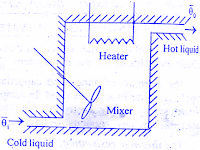#### For this system the equation for q0, C and R are obtained as follows,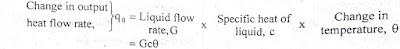#### Thermal capacitance, C = Mass, M x Specific heat of liquid, c = Mc#### In this thermal system,rate of change of temperature is directly proportional to change in heat input rate.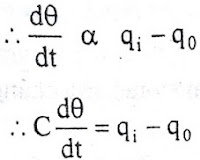The constant of proportionality is capacitance C of the system.

#### On substituting for q0 from equation (6) in equation (5) we get,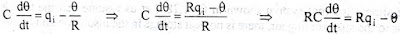#### θ(s)/Q1(s)  is the required transfer function of thermal system.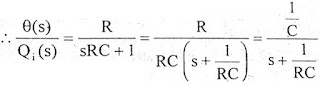READ HERE  Ohm's Law - Statement, Formula, Solved Examples